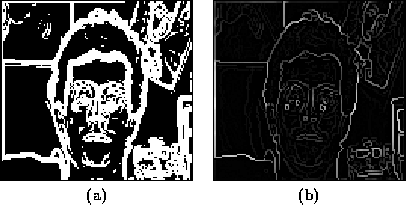Next: Multi-Scale Analysis Up: Edge Detection Previous: Deriche Edge Detection

## Edge Data Enhancement

Edge detection can be followed by further processing to enhance the output or to modify it. The techniques described below will be used on the output of the Sobel edge detection operation. The Sobel operation begins with an intensity image (Figure(a)) and produces a gradient magnitude map (Figure(b)) and an edge phase map (Figure(c)).Thresholding
If a binary (i.e., black and white) version of the gradient map is desired, it can be obtained by thresholding . All gradient values whose magnitudes are less than a threshold will be set to 0 and all gradient magnitudes greater than a threshold will be set to the maximum edge value (255). Thus, the maximum image contrast is compressed to 1 bit. One strategy for selecting the threshold in question is to first search for the strongest edge magnitude in the image region of interest. Once the peak edge magnitude is found, the threshold is computed as a percentage of this peak (e.g, 10%). A thresholded version of Figure(a) is Figure(a).
Non-Maximal Suppression
Non-maximal suppression nullifies the gradient value at a pixel if its gradient magnitude is non-maximal vis-a-vis neighbouring gradient magnitudes along the perpendicular to the pixel's gradient orientation. The result is a thinned edge map, as shown in Figure(b) where only the dominant edges are present.
Square Root of Edge Magnitude
It may be necessary to adjust the edge magnitudes returned by the Sobel operation to change the significance of the contrast in a computation. In other words, we may want to re-map the contrast levels to emphasize the weak edges in an image. In some subsequent processing steps, the magnitude of the edge at each point is replaced by the square-root of the magnitude to attenuate the effect of contrast.Next: Multi-Scale Analysis Up: Edge Detection Previous: Deriche Edge Detection
Tony Jebara
2000-06-23